# Balance the following equation in acidic conditions Phases are optional

Balance the following equation in acidic conditions Phases are optional.
S2O3 2- + Cu 2+ —> S4O6 2- + Cu+

Concepts and reason
Write the given unbalanced reaction in two half reactions separately.
Balance the atoms other than O and H on both sides. Then, balance the O and H.
Balance the electrons at last. Add the two half reactions to get the final balanced reaction.

Fundamentals
A balanced equation is an equation which contains all the reactants and products in a fixed ratio. A balanced equation has the following characteristics:

1. It should follow the law of conservation of mass.
2. The total number of different types of elements on reactant side should be equal to the product side.
3. Stoichiometric coefficients are put in front of the molecules on both the reactant and product side to balance a given reaction. The coefficients to be used are whole number or fractional number.

The unbalanced reaction is written as follows: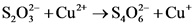The half reactions are written as follows:Explanation:
Separate the given unbalanced reaction in two half reactions involving the two species.

On balancing the S atoms, the reactions obtained are as follows: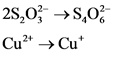Explanation:
Add a coefficient of 2 before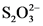to balance S atoms.
The Cu atoms are balanced.

On balancing the O atom and electrons, the reactions obtained are as follows: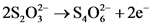Explanation:
The O atoms are balanced. Balance the charge using the electrons.

There are twoatoms with a charge of -2. So, total charge is -4.
The charge of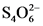is -2. So, add 2 electrons to the product side to balance the electrons in the first equation.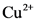has +2 charge andhas +1 charge. So, add one electron to the reactants in the second equation.
Multiply the second equation by 2 to equate the number of electrons in both the half reactions: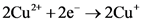The balanced reaction is written as follows: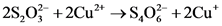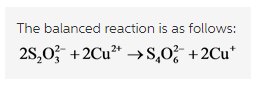Explanation:
The two reactions after balancing the electrons and atoms are as follows:Add both half reactions as follows:This is the final balanced reaction.